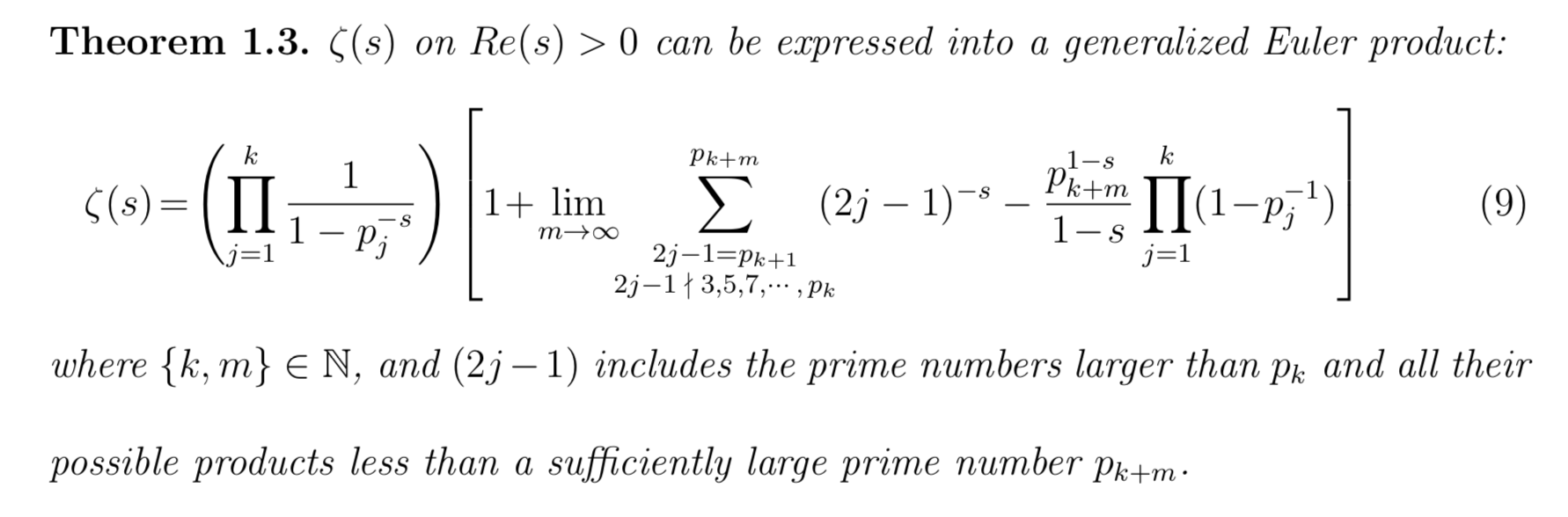# Product representations of the Riemann zeta function

The Riemann zeta function, initially defined as $$\zeta(s)=\sum_{n=1}^\infty \frac{1}{n^s}$$ for $\Re(s)>1$ also has infinite sum representations on larger domains. One such sum is $$\zeta(s)=\frac{1}{s-1}\sum_{n=1}^\infty \left(\frac{n}{(n+1)^s}-\frac{n-s}{n^s}\right),$$ defined for $\Re(s)>0$. Moreover, for any nonnegative integer $k$ there is a sum of this nature, analytic the half-plane $\Re(s) >-k$. I would like to know whether the Euler product representaiton $$\zeta(s)=\prod_{p \text{ prime}} \frac{1}{1-p^{-s}}, \; \Re(s)>1$$ also has some modified forms which converge on larger domains.

arXiv:1208.1440 has this generalized Euler product on $\Re(s)>0$: